# Matplotlib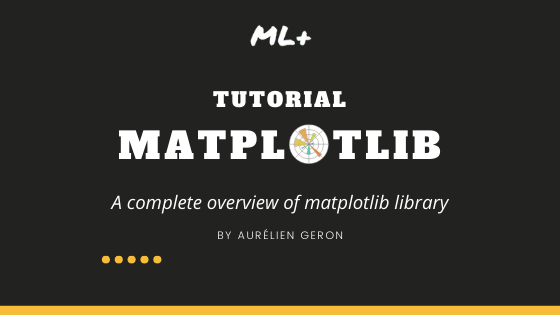## Matplotlib Tutorial – Principles of creating any plot with the Matplotlib library

Matplotlib is the most popular Python library to plot beautiful graphs. This tutorial guides you to grasp fundamental plotting through reproducible examples. Useful Posts: 1. Matplotlib Beginners Tutorial 2. Top 50 Matplotlib Plots for Data Analysis Overview This tutorial takes you through the following well-rounded concepts: 1. Plotting your first graph 2. Line style and …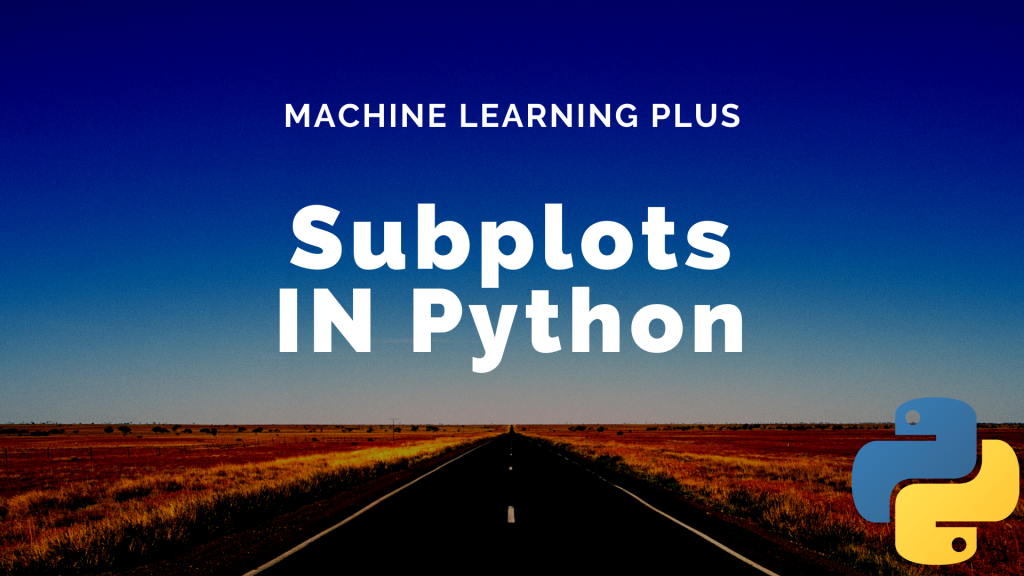## Subplots Python (Matplotlib)

Subplots mean groups of axes that can exist in a single matplotlib figure. subplots() function in the matplotlib library, helps in creating multiple layouts of subplots. It provides control over all the individual plots that are created. Matplotlib Subplots in Python CONTENTS Basic Overview axes() function add_axis() function Creating multiple grids in the same graph …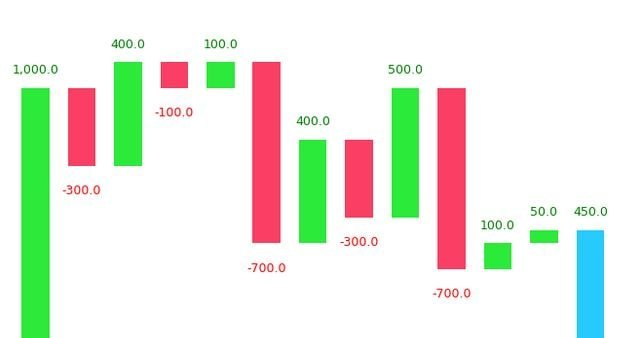## Waterfall Plot in Python

Waterfall chart is a 2D plot that is used to understand the effects of adding positive or negative values over time or over multiple steps or a variable. Waterfall chart is frequently used in financial analysis to understand the gain and loss contributions of multiple factors over a particular asset. Contents Introduction Simple Waterfall Plot …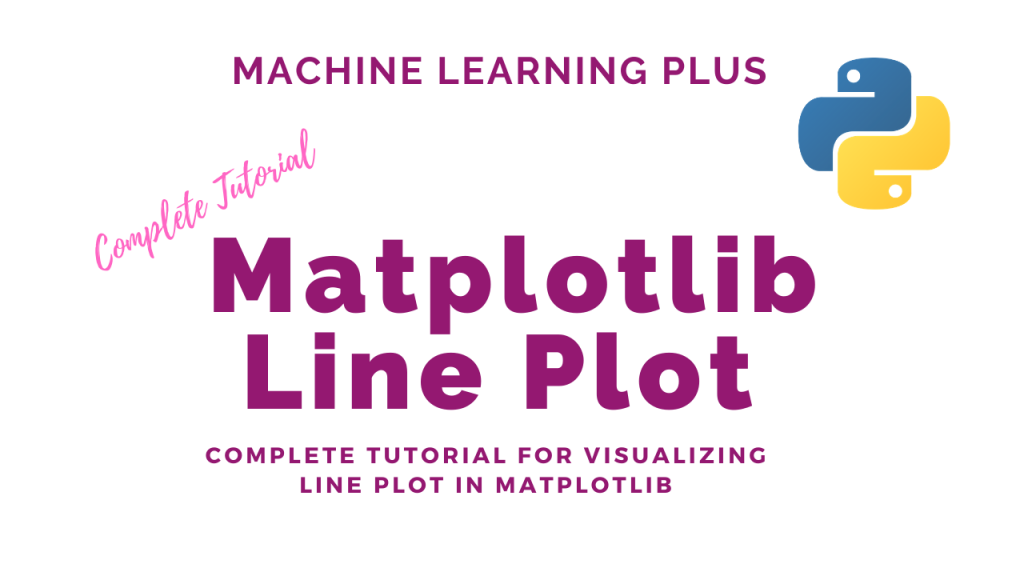## Matplotlib Line Plot

Line plot is a type of chart that displays information as a series of data points connected by straight line segments. A line plot is often the first plot of choice to visualize any time series data. Contents What is line plot? Simple Line Plot Multiple Line Plot in the same graph Creating a secondary …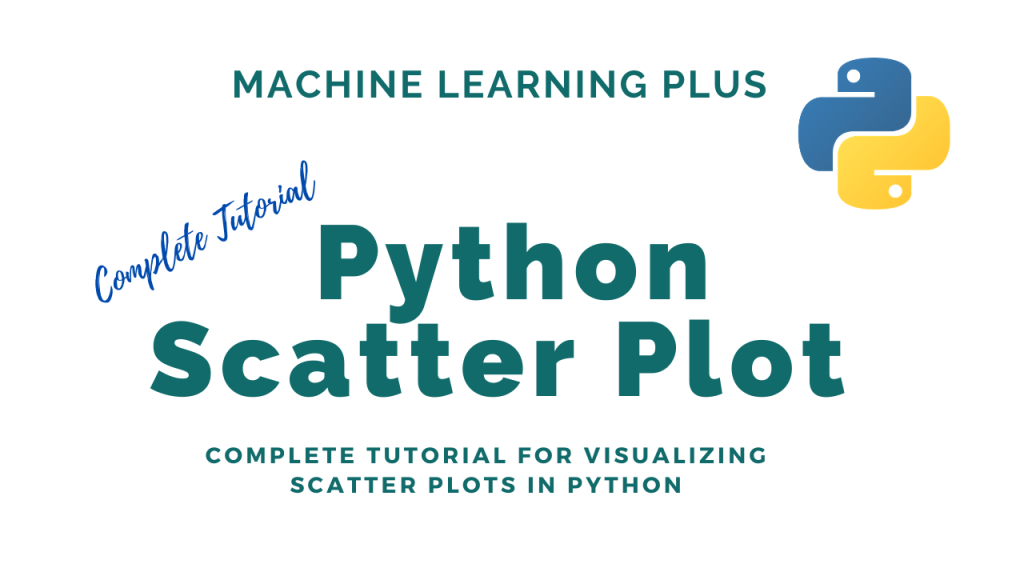## Python Scatter Plot

Scatter plot is a graph in which the values of two variables are plotted along two axes. It is a most basic type of plot that helps you visualize the relationship between two variables. Concept What is a Scatter plot? Basic Scatter plot in python Correlation with Scatter plot Changing the color of groups of …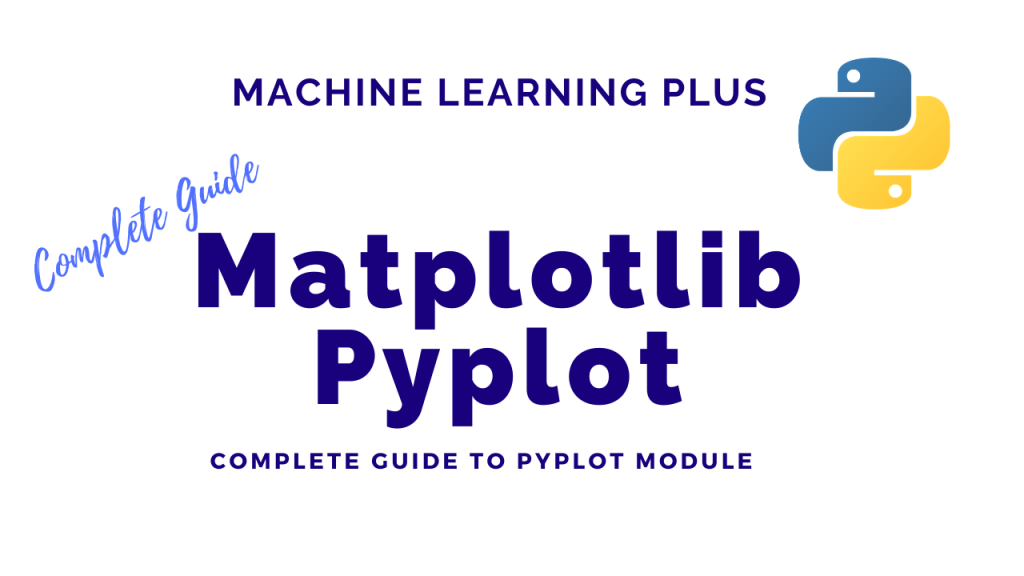## Matplotlib Pyplot

The pyplot object is the main workhorse of matplotlib library. It is through pyplot that you can create the figure canvas, various types of plots, modify and decorate them. Contents Pyplot: Basic Overview General Functions in pyplot Line plot Scatter plot Pie chart Histogram 2D Histograms Bar plot Stacked Barplot Boxplot Stackplot Time series plotting …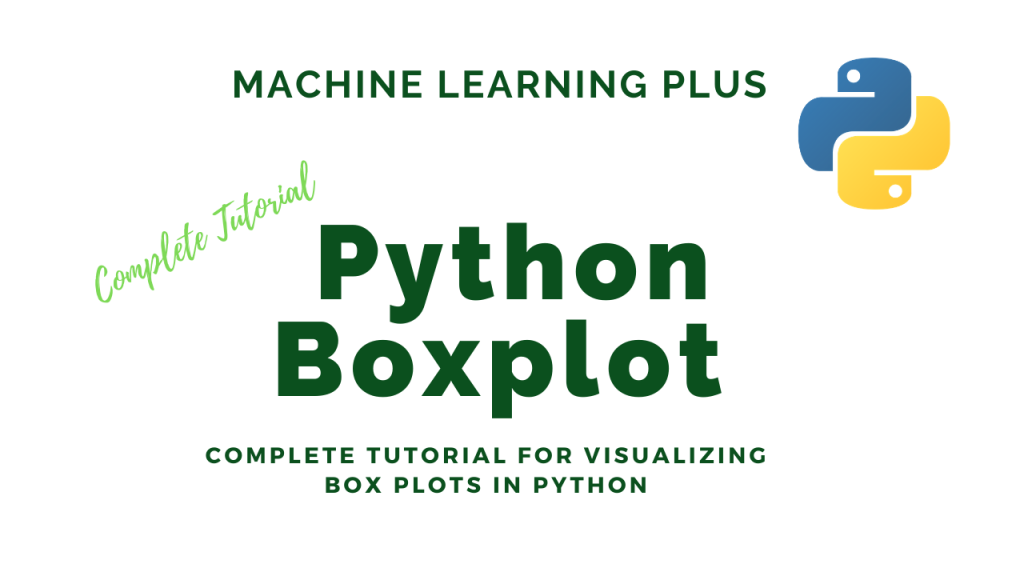## Python Boxplot

Boxplot is a chart that is used to visualize how a given data (variable) is distributed using quartiles. It shows the minimum, maximum, median, first quartile and third quartile in the data set. What is a boxplot? Box plot is method to graphically show the spread of a numerical variable through quartiles. From the below …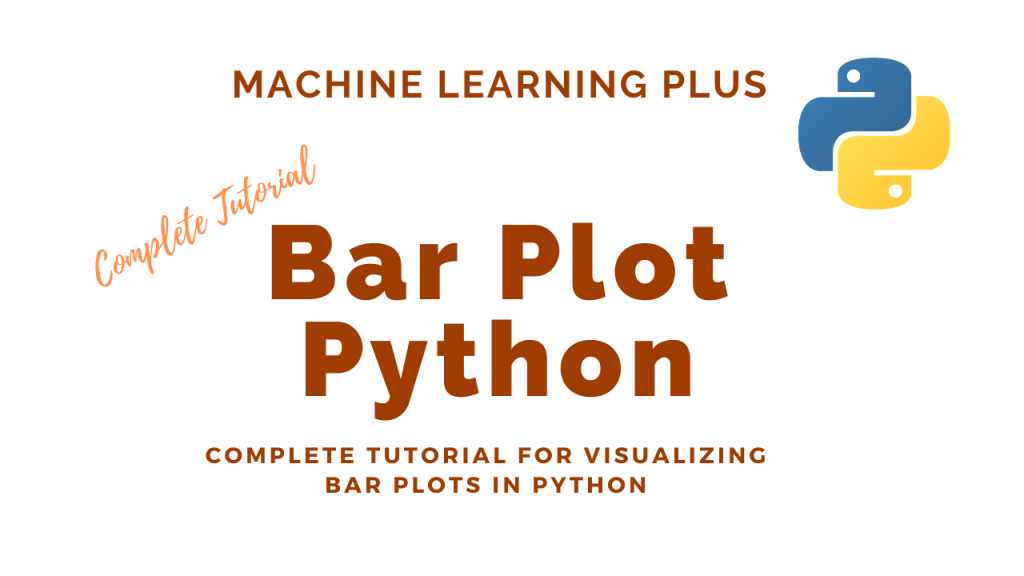## Bar Plot in Python

A bar plot shows catergorical data as rectangular bars with the height of bars proportional to the value they represent. It is often used to compare between values of different categories in the data. Content What is a barplot? Simple bar plot using matplotlib Horizontal barplot Changing color of a barplot Grouped and Stacked Barplots …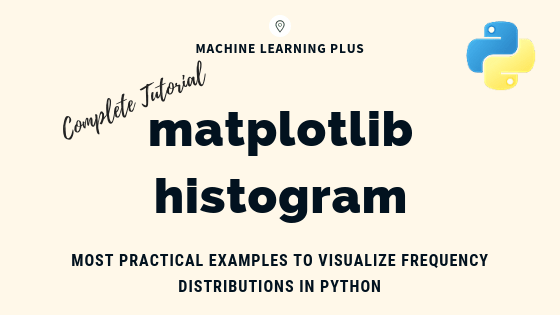## Matplotlib Histogram – How to Visualize Distributions in Python

Matplotlib histogram is used to visualize the frequency distribution of numeric array by splitting it to small equal-sized bins. In this article, we explore practical techniques that are extremely useful in your initial data analysis and plotting. Content What is a histogram? How to plot a basic histogram in python? Histogram grouped by categories in …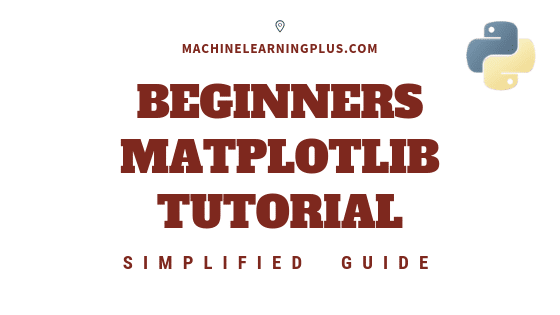## Matplotlib Tutorial – A Complete Guide to Python Plot with Examples

Until next time Until next time This tutorial explains matplotlib’s way of making plots in simplified parts so you gain the knowledge and a clear understanding of how to build and modify full featured matplotlib plots. 1. Introduction Matplotlib is the most popular plotting library in python. Using matplotlib, you can create pretty much any …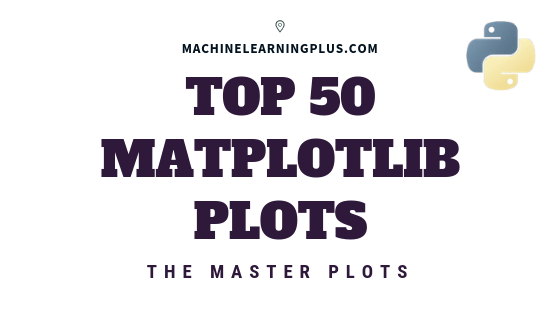## Top 50 matplotlib Visualizations – The Master Plots (with full python code)

A compilation of the Top 50 matplotlib plots most useful in data analysis and visualization. This list lets you choose what visualization to show for what situation using python’s matplotlib and seaborn library. Introduction The charts are grouped based on the 7 different purposes of your visualization objective. For example, if you want to picturize …Course Preview

## Machine Learning A-Z™: Hands-On Python & R In Data Science

### Free Sample Videos:#### Machine Learning A-Z™: Hands-On Python & R In Data Science#### Machine Learning A-Z™: Hands-On Python & R In Data Science#### Machine Learning A-Z™: Hands-On Python & R In Data Science#### Machine Learning A-Z™: Hands-On Python & R In Data Science